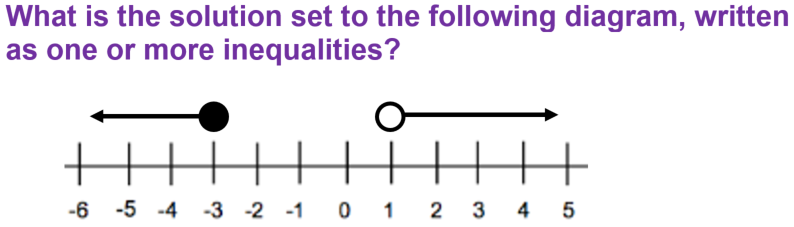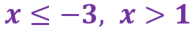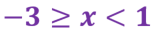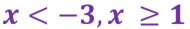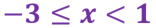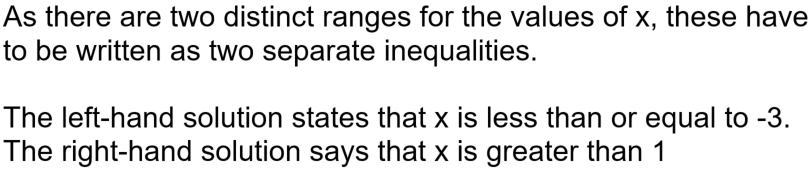See if you can get full marks in this informative quiz.

# Linear Inequalities (F)

If you thought the world of algebra was all about equations, think again! In a linear equation, the variable can only have one value. But in an inequality there could be many, many more possible solutions! This GCSE Maths quiz will test how well you understand the symbols, and if you can safely manipulate the algebra.

Inequalities can have a range of possible solutions, so we need a mathematical way to write this down. The range will have a number that is right at the boundary, or limit – sometimes this number is excluded from the solution, so we use the symbols < (less than) or > (greater than). At other times the number forms part of the solution, so we add an extra line to our symbols which acts like an equal sign. This gives us ≤ (less than or equal to) and ≥ (greater than or equal to). If you are given an inequality to solve, just stick with the symbol you are given. If you add or remove the ‘or equal to’ then you are changing the problem.

Have you ever wondered if inequalities exist in the real world? Of course they do! Think about the ages of people that are allowed to drive in Britain – you have to be 17 or older. This can be written as ‘age ≥ 17’. Or a soft-play area, in which you have to be 4 or older, but less than 12 to be able to play in it. As the range is between two limits, we can combine the inequalities and write this as 4 ≤ age < 12.

Next time you are out and about, see if you can find any more situations that you can write as an inequality!

Question 1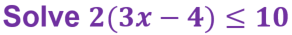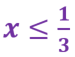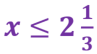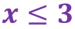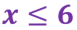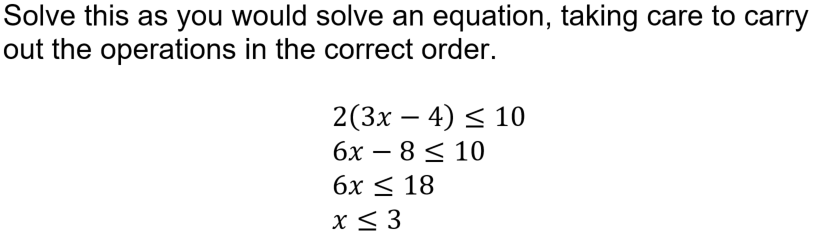Question 2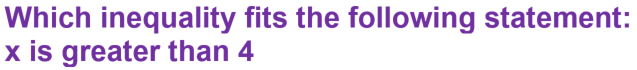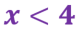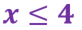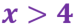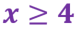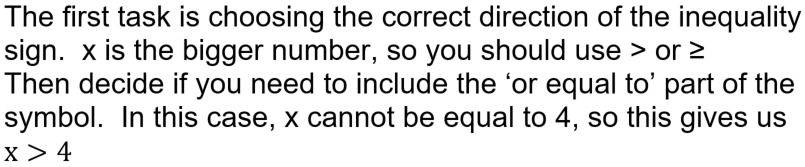Question 3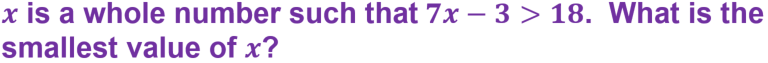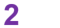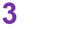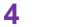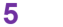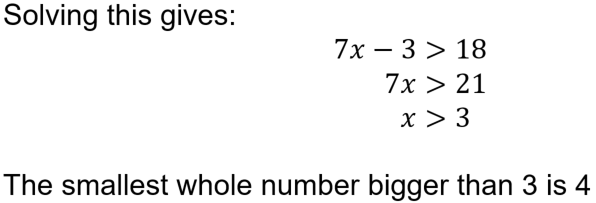Question 4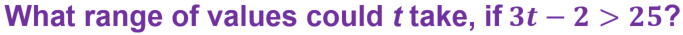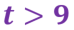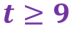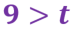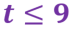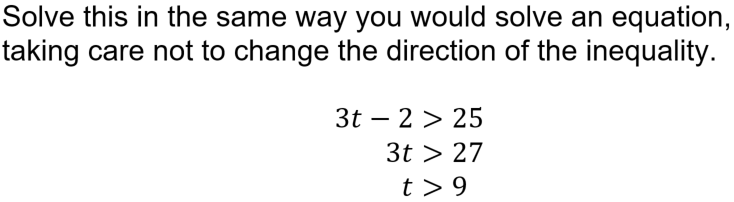Question 5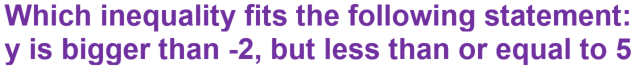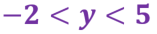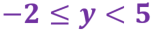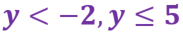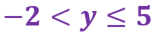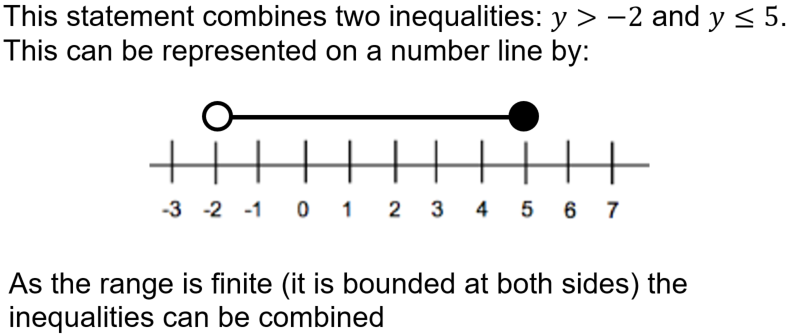Question 6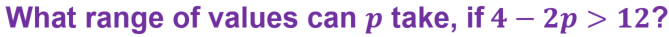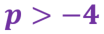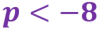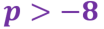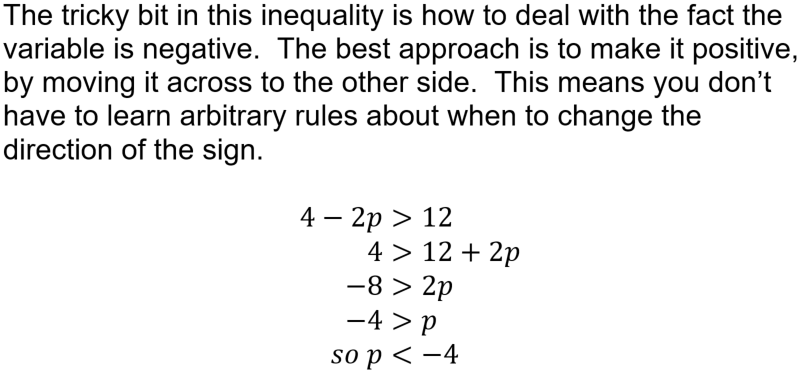Question 7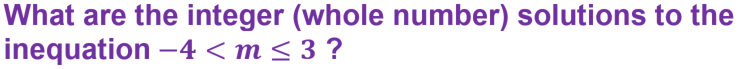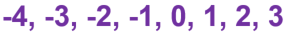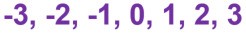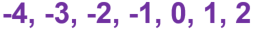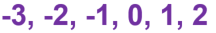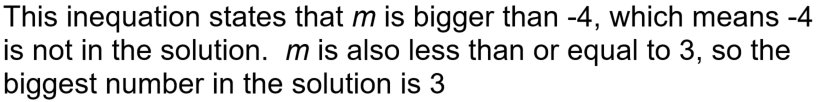Question 8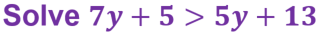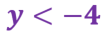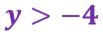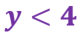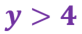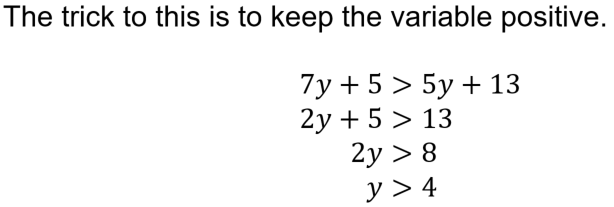Question 9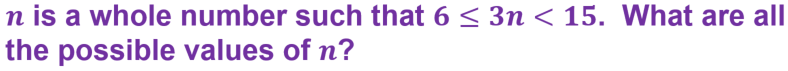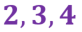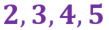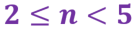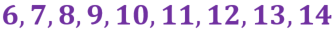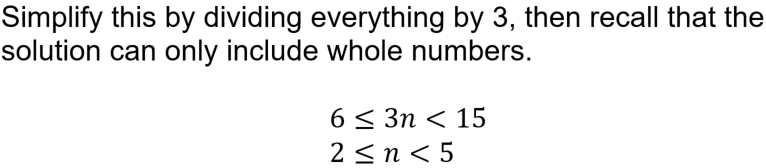Question 10